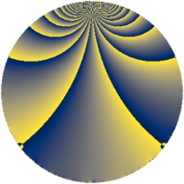# Properties

 Label 98.2.gLevel $98$ Weight $2$ Character orbit 98.g Rep. character $\chi_{98}(9,\cdot)$ Character field $\Q(\zeta_{21})$ Dimension $48$ Newform subspaces $2$ Sturm bound $28$ Trace bound $2$

# Related objects

## Defining parameters

 Level: $$N$$ $$=$$ $$98 = 2 \cdot 7^{2}$$ Weight: $$k$$ $$=$$ $$2$$ Character orbit: $$[\chi]$$ $$=$$ 98.g (of order $$21$$ and degree $$12$$) Character conductor: $$\operatorname{cond}(\chi)$$ $$=$$ $$49$$ Character field: $$\Q(\zeta_{21})$$ Newform subspaces: $$2$$ Sturm bound: $$28$$ Trace bound: $$2$$ Distinguishing $$T_p$$: $$3$$

## Dimensions

The following table gives the dimensions of various subspaces of $$M_{2}(98, [\chi])$$.

Total New Old
Modular forms 192 48 144
Cusp forms 144 48 96
Eisenstein series 48 0 48

## Trace form

 $$48q + 4q^{4} - 14q^{6} - 14q^{9} + O(q^{10})$$ $$48q + 4q^{4} - 14q^{6} - 14q^{9} - 18q^{11} + 2q^{15} + 4q^{16} - 14q^{17} - 4q^{18} - 14q^{19} - 14q^{20} - 6q^{22} - 50q^{23} - 4q^{25} - 14q^{26} - 42q^{27} + 2q^{29} - 8q^{30} - 14q^{35} - 14q^{36} - 4q^{37} + 28q^{38} + 16q^{39} + 28q^{40} + 56q^{41} - 42q^{42} + 16q^{43} + 24q^{44} + 14q^{45} + 76q^{46} - 28q^{47} + 112q^{49} + 24q^{50} + 92q^{51} - 14q^{52} - 34q^{53} + 84q^{54} + 42q^{55} + 28q^{56} - 10q^{57} - 12q^{58} + 42q^{59} + 20q^{60} + 28q^{62} - 42q^{63} - 8q^{64} - 28q^{65} - 12q^{67} - 28q^{68} - 28q^{69} - 14q^{70} + 6q^{71} - 4q^{72} - 42q^{73} - 26q^{74} - 84q^{75} - 42q^{77} - 24q^{78} - 20q^{79} - 14q^{80} + 10q^{81} - 84q^{83} - 42q^{84} + 16q^{85} - 54q^{86} + 98q^{87} - 18q^{88} - 56q^{89} - 42q^{90} - 14q^{91} + 16q^{92} + 34q^{93} - 70q^{94} + 72q^{95} - 28q^{97} - 28q^{98} - 120q^{99} + O(q^{100})$$

## Decomposition of $$S_{2}^{\mathrm{new}}(98, [\chi])$$ into newform subspaces

Label Dim. $$A$$ Field CM Traces $q$-expansion
$$a_2$$ $$a_3$$ $$a_5$$ $$a_7$$
98.2.g.a $$24$$ $$0.783$$ None $$-2$$ $$7$$ $$0$$ $$0$$
98.2.g.b $$24$$ $$0.783$$ None $$2$$ $$-7$$ $$0$$ $$0$$

## Decomposition of $$S_{2}^{\mathrm{old}}(98, [\chi])$$ into lower level spaces

$$S_{2}^{\mathrm{old}}(98, [\chi]) \cong$$ $$S_{2}^{\mathrm{new}}(49, [\chi])$$$$^{\oplus 2}$$